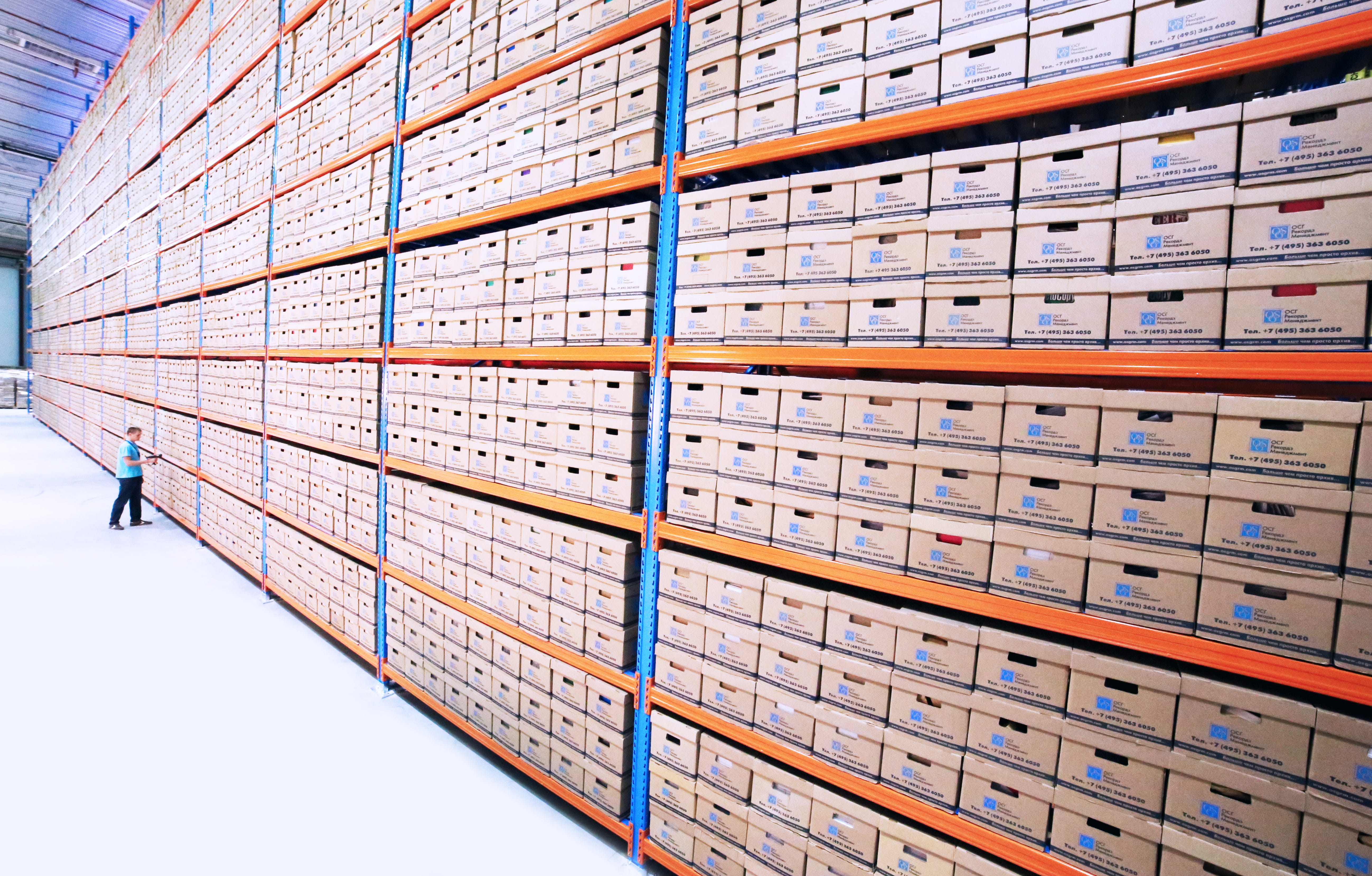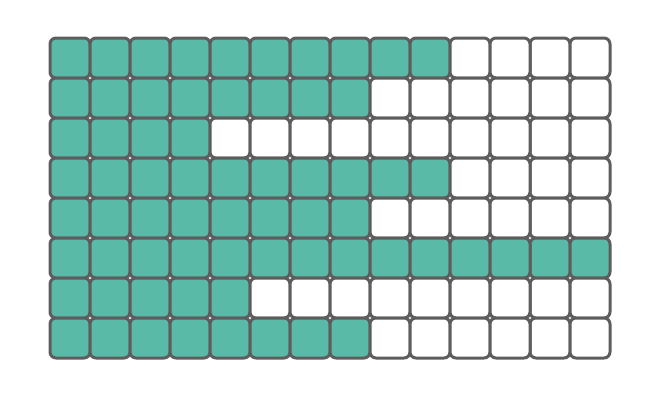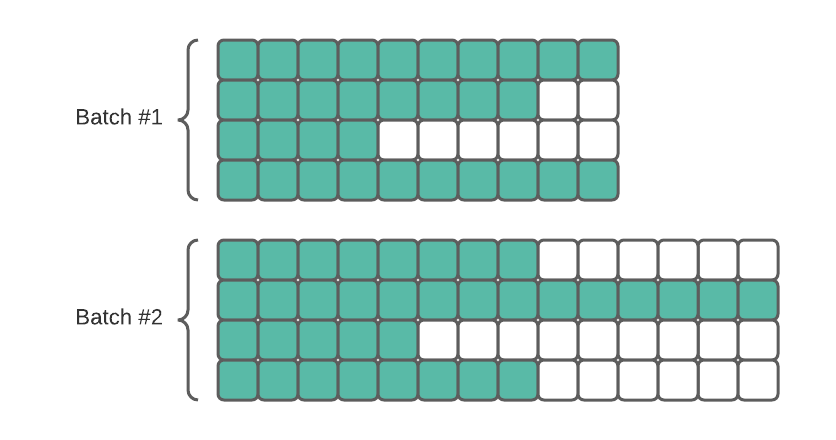# Understand collate_fn in PyTorch

## A beginner-friendly tutorial on how to create batches in PyTorch and how to modify default behavior for our needs.

The `DataLoader` is one of the most commonly used classes in PyTorch. Also, it is one of the first you learn. This class has a lot of parameters (14), but most likely, you will use about three of them (`dataset`, `shuffle`, and `batch_size`). Today I’d like to explain the meaning of `collate_fn`— which I found confusing for beginners in my experience. We will briefly explore how PyTorch creates batch and see how we can modify default behavior for our needs.Photo by Nana Smirnova on Unsplash

## Batch creation process

One of the first pieces of information in every deep learning course is that we perform training/inferencing in batches. Most of the time, a batch is just a number of stacked examples. But in some cases, we would like to modify how it is created.

First things first, let’s investigate what happens in the default case. Assume we have the following toy dataset. It contains four examples, three features each.

``````import torch
import numpy as np

data = np.array([
[0.1, 7.4, 0],
[-0.2, 5.3, 0],
[0.2, 8.2, 1],
[0.2, 7.7, 1]])
print(data)
``````

If we ask a loader for a batch, we will see the following (note that I set `shuffle=False` to eliminate randomness):

``````loader = DataLoader(data, batch_size=2, shuffle=False)
print(batch)

# tensor([[ 0.1000,  7.4000,  0.0000],
#         [-0.2000,  5.3000,  0.0000]], dtype=torch.float64)
``````

No surprise, but let’s formalize what was has been done:

1. Loader selected 2items from the dataset.

2. Those items were converted into a tensor (2 items of size 3).

3. A new tensor was created (2x3) and returned.

Default setup also allows us to use dictionaries. Let’s see an example:

``````from pprint import pprint
# now dataset is a list of dicts
dict_data = [
{'x1': 0.1, 'x2': 7.4, 'y': 0},
{'x1': -0.2, 'x2': 5.3, 'y': 0},
{'x1': 0.2, 'x2': 8.2, 'y': 1},
{'x1': 0.2, 'x2': 7.7, 'y': 10},
]
pprint(dict_data)
# [{'x1': 0.1, 'x2': 7.4, 'y': 0},
# {'x1': -0.2, 'x2': 5.3, 'y': 0},
# {'x1': 0.2, 'x2': 8.2, 'y': 1},
# {'x1': 0.2, 'x2': 7.7, 'y': 10}]

pprint(batch)
# {'x1': tensor([ 0.1000, -0.2000], dtype=torch.float64),
#  'x2': tensor([7.4000, 5.3000], dtype=torch.float64),
#  'y': tensor([0, 0])}
``````

The loader was smart enough to correctly repack data from a list of dicts. This capability is handy when your data is in JSONL format (which I personally prefer over CSV).

### Custom collate function

If default collation is so smart, why might we need to create a custom one? Default one has a significant limitation — batch data must be in the same dimension. Imagine we have an NLP task, and the data is tokenized text.

``````# values are token indices but it does not matter - it can be any kind of variable-size data
nlp_data = [
{'tokenized_input': [1, 4, 5, 9, 3, 2],
'label':0},
{'tokenized_input': [1, 7, 3, 14, 48, 7, 23, 154, 2],
'label':0},
{'tokenized_input': [1, 30, 67, 117, 21, 15, 2],
'label':1},
{'tokenized_input': [1, 17, 2],
'label':0},
]
``````

It will not work and raise an Error:

``````/usr/local/lib/python3.7/dist-packages/torch/utils/data/_utils/collate.py in default_collate(batch)
80         elem_size = len(next(it))
81         if not all(len(elem) == elem_size for elem in it):
---> 82             raise RuntimeError('each element in list of batch should be of equal size')
83         transposed = zip(*batch)
84         return [default_collate(samples) for samples in transposed]

RuntimeError: each element in list of batch should be of equal size
``````

The error message says that it is impossible to create a non-rectangular tensor. BTW: See that the error is the `default_collate` function.

What can we do? There are two solutions:

1. Pad the whole dataset to the longest example.

2. Pad dynamically during batch creation.

The first solution might seem more straightforward — just expand all examples to the longest one. But there is an issue — we will waste memory and computing power (they are expensive on GPU!) for processing padding, which does not influence the result. It is especially painful if we have a few long sequences in the data, and most of them are relatively short. In such a case, we are mostly process padding instead of data!If we pad the whole dataset to the longest sequence, there is a lot of wasted space!

An alternative is to pad the data on the fly. When samples for the batch are selected, we pad only them to the longest one. If we additionally order the data by length, the padding will be minimal. If there are a few very long sequences, they will only influence their batches- not the whole dataset.Per-batch padding reduces the number of unnecessary spaces used.

Okay, but how to implement it? Just create a custom `collate_fn`. It is simple, I promise ;)

``````from torch.nn.utils.rnn import pad_sequence #(1)

def custom_collate(data): #(2)
inputs = [torch.tensor(d['tokenized_input']) for d in data] #(3)
labels = [d['label'] for d in data]

labels = torch.tensor(labels) #(5)

return { #(6)
'tokenized_input': inputs,
'label': labels
}

pprint(batch1)
pprint(batch2)

# {'label': tensor([0, 0]),
#  'tokenized_input': tensor([
#   [  1,   4,   5,   9,   3,   2,   0,   0,   0],
#   [  1,   7,   3,  14,  48,   7,  23, 154,   2]
# ])}

# {'label': tensor([1, 0]),
#  'tokenized_input': tensor([
#   [  1,  30,  67, 117,  21,  15,   2],
#   [  1,  17,   2,   0,   0,   0,   0]])}
``````

Step by step:

2. Collate function takes a single argument — a list of examples. In this case, it will be a list of dicts, but it also can be a list of tuples, etc. — depending on the dataset.

3. As data comes if format “list of dicts” we need to traverse it and create a separate list for all inputs and labels. In the meantime, tokenized_input is converted to a 1-D tensor (it was a list of ints).

5. As labels were a list of ints, we converted it into a tensor.

6. Return formatted batch.

7. Set our custom function in the loader.

As we can see, the batch is in the same format as for default collation with a dictionary. We clearly see that amount of padding is only minimal.

## Conclusion

Creating a custom collation maybe is not the most common task, but you definitely need to know how to do it.

## Exercise

If you are using 🤗 Transformers, try to write a collator that will tokenize the data on the fly.